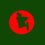# Expected Value

A box contains 25 balls which are numbered from $1$ to $25$. I took a ball.What is the expected number that the ball have?Note by Kiriti Mukherjee
7 years ago

This discussion board is a place to discuss our Daily Challenges and the math and science related to those challenges. Explanations are more than just a solution — they should explain the steps and thinking strategies that you used to obtain the solution. Comments should further the discussion of math and science.

When posting on Brilliant:

• Use the emojis to react to an explanation, whether you're congratulating a job well done , or just really confused .
• Ask specific questions about the challenge or the steps in somebody's explanation. Well-posed questions can add a lot to the discussion, but posting "I don't understand!" doesn't help anyone.
• Try to contribute something new to the discussion, whether it is an extension, generalization or other idea related to the challenge.

MarkdownAppears as
*italics* or _italics_ italics
**bold** or __bold__ bold
- bulleted- list
• bulleted
• list
1. numbered2. list
1. numbered
2. list
Note: you must add a full line of space before and after lists for them to show up correctly
paragraph 1paragraph 2

paragraph 1

paragraph 2

[example link](https://brilliant.org)example link
> This is a quote
This is a quote
    # I indented these lines
# 4 spaces, and now they show
# up as a code block.

print "hello world"
# I indented these lines
# 4 spaces, and now they show
# up as a code block.

print "hello world"
MathAppears as
Remember to wrap math in $$ ... $$ or $ ... $ to ensure proper formatting.
2 \times 3 $2 \times 3$
2^{34} $2^{34}$
a_{i-1} $a_{i-1}$
\frac{2}{3} $\frac{2}{3}$
\sqrt{2} $\sqrt{2}$
\sum_{i=1}^3 $\sum_{i=1}^3$
\sin \theta $\sin \theta$
\boxed{123} $\boxed{123}$

Sort by:

The answer is $13$. [provided that all the balls have an equal chance of being selected]

The expected value of a random variable is the weighted average of all possible outcomes.

In your example the probability of a ball being selected is $\frac{1}{25}$. So the expected value would be:

$1(\frac{1}{25})+2(\frac{1}{25})+3(\frac{1}{25})+4(\frac{1}{25})+\cdots + 23(\frac{1}{25})+ 24(\frac{1}{25})+25(\frac{1}{25})$

$=13$.

Hope this helps!

- 7 years ago

You might want to read this: Expected Value | Brilliant

- 7 years ago

You probably wanted to post this link.

- 7 years ago

Thanks for pointing that out, I've updated it.

- 7 years ago

If I choose a ball again with out replace the first ball .What is the expected value of the second ball?

- 7 years ago

We are not here to solve problems for you. Mursalin H. told you how to solve the first problem, and if you understand it completely, you should be able to solve this one yourself. I have also provided you with the Brilliant post about the expected value, which will help you understand the concept.

- 7 years ago

Just call me Mursalin. Mursalin H. sounds really odd! :)

- 7 years ago

wen all are equiprobable how can one of them(13) have more chances of appearing

- 7 years ago